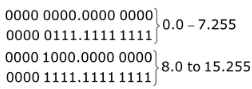# GATE Solved Paper 2020 - GATE 2020

• A

II and III only• B

I and II only• C

III and IV only• D

I and IV only• Option : A
• Explanation :
No. of host = 1500
No. of host bits = [log2 1500] = 11 bits
∴ Total possible hosts = 211 = 23 × 28
Range of addresses is = 232 – n
211 = 232-n0.0 to 7.255
8.0 to 15.255
16.0 to 23.255
:
64.0 to ...
:
104.0 to ...
∴ The possible IP addresses are 202.61.64.0/21 & 202.61.104.0/21

• A

L2 and L3 only• B

L1 and L3 only• C

L1, L3 and L4 only• D

L2, L3 and L4 only• Option : C
• Explanation :
L1 L(m) = ϕ ⇒ emptiness problem of TM.
TM is undecidable under emptiness.
L2 = where a TM visits a particular state in finite steps in decidable, as we can do this with UTM.
L3 = L(m) is non-Recursive,Clearly from the diagram
L(A) ⇒ non recursive language accepted by TM
L(B) ⇒ non-recursive language not accepted by TM.
∴ it is a non-trivial property, hence undecidable.
L4 = Undecidable problem using rice-theorem.
Hence, L1, L3, and L4 are undecidable.

• A

21• B

15• C

13• D

23• Option : C
• Explanation :
Given Hash Function ⇒ h1(k) = k mod 23
h2(k) = 1 + (k mod 19)
Table size = 23
Key = 90
h1(k) = 90 mod 23 ≡ 21
h2(k) = 1 + 90 mod 19 ≡ 1 + 14 = 15
Double hashing, (h1(k) + i.h2(k)) mod 23
Asked for probe 1, put i = 1
(21 + 1. (15)) mod 23
36 mod 23 = 13

• A

5• B

6• C

4• D

3• Option : B
• Explanation :
In non-persistent HTTP, each packet takes 2 RTT (Round trip Time): one for TCP connection, one or HTTP Text (Image file As, it is given text and 5 images that totals 6 objects.)
So, it takes 12 RTT in total. But,
12 RTT includes 6 HTTP connections + 6TCP connections.
So, the minimum number of TCP connections required is 6.

• A

1• B

5• C

7• D

35• Option : C
• Explanation :
According to Lagrange’s theorem, state that for any finite group G, the order (number of element) of every subgroup t1 of G divides the order of G. therefore, possible subgroup of group of 35 elements.
{1, 5, 7, 35}
Related Quiz.
GATE 2020